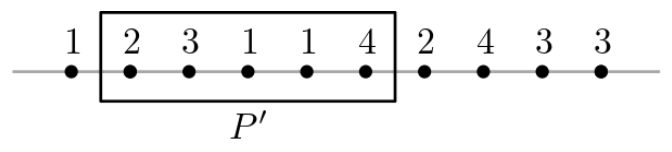시간 제한메모리 제한제출정답맞힌 사람정답 비율
1 초 (추가 시간 없음) 1024 MB148998368.595%

## 문제

Let $P$ be a set of $n$ points on the $x$-axis and each of the points is colored with one of the colors $1, 2, \dots , k$. For each color 𝑖 of the 𝑘 colors, there is at least one point in $P$ which is colored with $i$. For a set $P'$ of consecutive points from $P$, if both $P'$ and $P \backslash P'$ contain at least one point of each color, then we say that $P'$ makes a double rainbow. See the below figure as an example. The set 𝑃 consists of ten points and each of the points is colored by one of the colors $1$, $2$, $3$, and $4$. The set $P'$ of the five consecutive points contained in the rectangle makes a double rainbow.Given a set $P$ of points and the number $k$ of colors as input, write a program that computes and prints out the minimum size of $P'$ that makes a double rainbow.

## 입력

Your program is to read from standard input. The input starts with a line containing two integers $n$ and $k$ ($1 ≤ k ≤ n ≤ 10,000$), where $n$ is the number of the points in $P$ and $k$ is the number of the colors. Each of the following $n$ lines consists of an integer from $1$ to $k$, inclusively, and the $i$-th line corresponds to the color of the $i$-th point of $P$ from the left.

## 출력

Your program is to write to standard output. Print exactly one line. The line should contain the minimum size of $P'$ that makes a double rainbow. If there is no such $P'$, print 0.

## 예제 입력 1

10 4
1
2
3
1
1
4
2
4
3
3


## 예제 출력 1

5


## 예제 입력 2

6 3
1
1
2
2
3
3


## 예제 출력 2

0